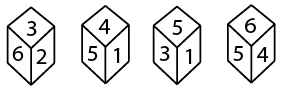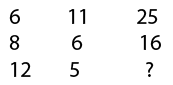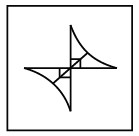# SSC CPO 20 March 2016 Afternoon Shift

Instructions

For the following questions answer them individually

Question 1

# Four portions of a cube are shown below. Identify the number at the bottom when top is 6 ?Instructions

In each of the following questions, select the missing number from the given responses.

Question 2

Question 3

Question 4

Question 5

#Instructions

In each of the following questions, from the given answer figures, select the one is which the question figure is hidden/embedded.

Question 6

Question 7

#Instructions

In each of the following questions, which answer figure will complete the pattern in the question figure ?

Question 8

Question 9

# Fig

Instructions

In the each of the following questions, find the odd word/letters/number pair from the given alternatives.